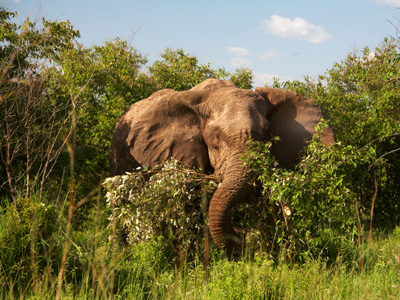An African elephant exerts pressure on the ground on which it stands. That pressure is measured in pascals.

# Pressure 1

This Science quiz is called 'Pressure 1' and it has been written by teachers to help you if you are studying the subject at middle school. Playing educational quizzes is a fabulous way to learn if you are in the 6th, 7th or 8th grade - aged 11 to 14.

It costs only \$12.50 per month to play this quiz and over 3,500 others that help you with your school work. You can subscribe on the page at Join Us

Pressure is an effect which occurs when a force is applied on a surface. Pressure is the amount of force acting on a unit area. In order to calculate pressure, we need to know the force applied over a known area.

Try this Science quiz and see how well you understand pressure.

1.
What is force measured in?
Joules
Kilograms
Newtons
Pascals
2.
What is pressure measured in?
Joules
Kilograms
Newtons
Pascals
1 Pa = 1N/1m2
3.
If pressure is measured in pascals, Pa, and force in newtons, N, then area is measured in .......
cm2
km2
m2
mm2
4.
If pressure increases then .......
force and area are doubled
force and area are halved
force increases or area decreases
force increases or area increases
This is because pressure = force/area
5.
A box weighs 100N. The area of the box on the ground is 20m2. What is the pressure of the box on the ground?
0.02Pa
5Pa
50Pa
2,000Pa
Pressure = force/area = 100N/20m2 = 5Pa
6.
A box weighs 60N. The dimensions of the base are 3m by 2m. What is the pressure of the box on the ground?
10Pa
12Pa
20Pa
30Pa
Pressure = force/area, area = 3m x 2m = 6m2, pressure = 60/6 = 10Pa
7.
Another box weighs 60N. The base dimensions are 2m by 1m. What is the pressure on the ground?
6Pa
20Pa
30Pa
60Pa
Area of base = 2 x 1 = 2m2 so pressure = 60/2 = 30Pa
8.
A student weighs 600N. The area of one foot is 0.01m2. If she stands on two feet what is the pressure on the ground?
300Pa
6,000Pa
30,000Pa
60,000Pa
Area of two feet = 0.01 x 2 = 0.02 pressure = 600/0.02
9.
The dimensions of the base of a box are 80cm by 100cm. It weighs 400N. What is the pressure on the ground?
50Pa
80Pa
400Pa
500Pa
80cm = 0.8m, 100cm = 1m, area = 0.8 x 1 = 0.8m2, pressure = 400/0.8 = 500Pa
10.
The size of an African elephant's foot is 40cm by 50cm. It weighs 64,000N. When standing on the ground, what is the pressure it exerts?
40,000Pa
80,000Pa
240,000Pa
300,000Pa
Area of foot = 40cm x 50cm = 0.4m x 0.5m = 0.2m2, area of four feet = 0.8m2, pressure = 64,000/0.8
Author:  Sue Davison